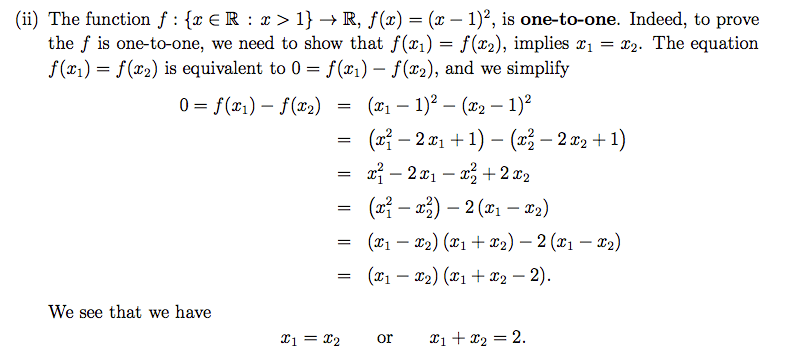# Proof for one-to-one function

Please may someone explain the last step of this proof for me? I can't see how it's done algebraically. Where does the second (x1-x2) go? Is it a mistake?I've tried multiplying out -2 and everything else I could think of but to no avail.

Thank you.

Hi chickensagan.
There is no error, this is just factorization.
maybe you are being intimidated by the (x2-x1) expression, just call it 'a'. and call 'x1+x2' 'b'
so in the last step you have a.b - 2.a = a(b-2)

Cheers...

Thanks for the reply Oli4, but I still don't get it.
I don't understand how they factorise it when both sides don't have 2 as a common factor, and even if that happened how does the -2 end up inside the bracket?

what is being factorized is the term (x2-x1) or the term 'a' in my simplified version.
a*b+a*2=a*(b+2) <- is this problematic for you ? just check it by doing the expansion.
in this case it is a*b-a*2=a*(b-2)
with a being x2-x1 and b being x2+x1

CAF123
Gold Member
Perhaps more simply:$$(x_1 - x_2)(x_1 + x_2) - 2(x_1 - x_2) = (x_1 - x_2)[(x_1 + x_2) - 2].$$Using the [,] might make you see it easier - I just used a common factor of ##(x_1 - x_2)##.